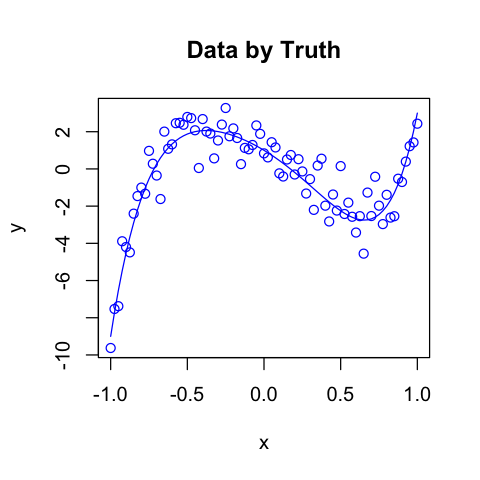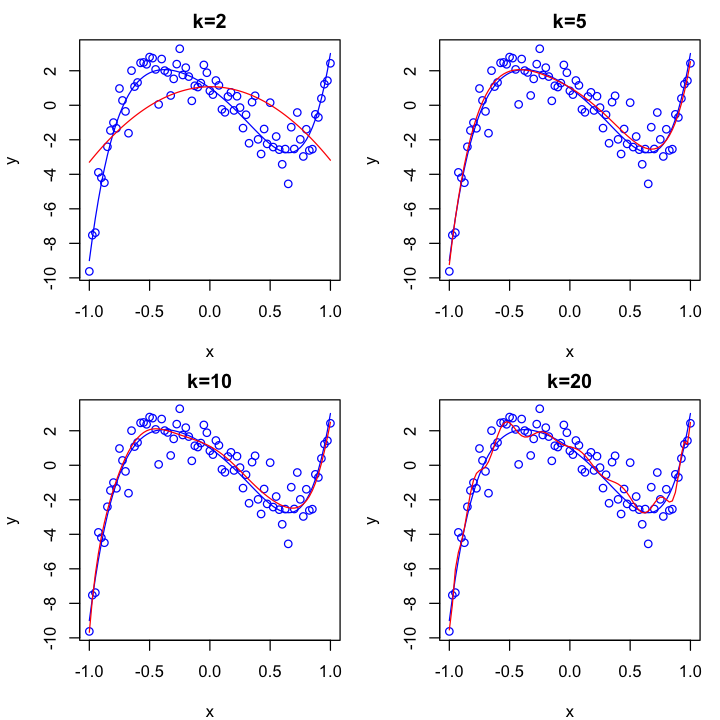# The Akaike Information Criterion¶

Nov. 5, 2018 $\newcommand{\bs}{\boldsymbol}$ $\newcommand{\argmin}{\underset{\bs{#1}}{\text{arg min}}\,}$ $\newcommand{\argmax}{\underset{\bs{#1}}{\text{arg max}}\,}$ $\newcommand{\tr}{^{\top}}$ $\newcommand{\norm}{\left|\left|\,#1\,\right|\right|}$ $\newcommand{\given}{\,|\,}$ $\DeclareMathOperator{\E}{\mathbb{E}}$

The Akaike information criterion (AIC) is an estimator of the relative strength of statistical models given a set of observed data. As mentioned in my previous posts, statistics is concerned with estimating the unknown data generating distribution $p(y)$ by assuming a modeling distribution $p_{\text{model}}(y \given \bs{\theta})$, where $\bs{\theta}$ represent the parameters of the model. The model can be as simple as a normal distribution centered at $\bs{x}\tr \bs{\theta}$, i.e.,

$$p_{\text{model}}(y\given \bs{x}, \bs{\theta}) = \left(\frac{1}{2\pi \sigma}\right)^{\frac{1}{2}} \text{exp}\left(-\frac{1}{2\sigma} (y-\bs{x}\tr\bs{\theta})^2 \right).$$

In this case we have the ordinary multiple linear regression. It can also be as sophisticated as a deep feed-forward neural network, where the modeling distribution is a composition of many linear and nonlinear activation functions, representing the input, hidden, and output layers of the neural network.

Since there is no way of knowing the exact data generating distribution $p$, any model we propose will result in a loss of information. The AIC measures the relative information loss by a given model. The AIC is named after the statistician Hirotugu Akaike, and is one of the most widely used model selection techniques (another important technique is cross-validation).

## Definition¶

Suppose $q_1(y\given \bs{\theta}_1)$ and $q_2(y \given \bs{\theta}_2)$ are two modeling distributions, where $\bs{\theta}_1\in \mathbb{R}^{k_1}$ and $\bs{\theta}_2\in \mathbb{R}^{k_2}$. Denote by $p(y)$ the data generating distribution, then we can compute the Kullback-Liebler divergence $D_{\text{KL}}(p\,||\,q_i)$ for $i=1,2$ as

\begin{aligned} D_{\text{KL}}(p\,||\,q_i) &= \E_{y\sim p} \left[\log \frac{p(y)}{q_i(y\given \bs{\theta}_i)} \right] \\ &= \underbrace{- \int p(y) \log q_i(y\given \bs{\theta}_i)\, dy}_{\text{Cross-entropy}} + \underbrace{\int p(y) \log p(y)\, dy}_{\text{Entropy}}. \end{aligned}\tag{1}

Since the entropy is not dependent on $i$, we choose the best model by minimizing the cross-entropy:

$$K_i = -\int p(y) \log q_i(y\given \bs{\widehat{\theta}}_i)\, dy. \tag{2}$$

where $\bs{\widehat{\theta}}_i$ is the maximum likelihood estimator for $\bs{\theta}_i$ given an empirical distribution $\widehat{p}(y)$ from the observed data $D^n = \{y^{(i)} : i=1,...,n\}$, computed as

$$\bs{\widehat{\theta}}_i = \argmin{\theta_i \in \Theta_i} D_{KL}(\widehat{p}\, ||\, q_i). \tag{3}$$

Our goal is to estimate $K_i$. At first we are tempted to use $\widehat{p}$ to estimate $p$, yielding

$$\overline{K}_i = -\frac{1}{n} \sum_{j=1}^n \log p(y^{(j)} \given \bs{\widehat{\theta}}_i) = -\frac{1}{n}l_i(\bs{\widehat{\theta}_i}) \tag{4}$$

where $l_i(\bs{\theta}_i)$ is the log-likelihood function for model $i$. However, this quantity is very biased because the data $D^n$ is used both to estimate $\widehat{\bs{\theta}}_i$ and also the integral. To correct for the bias, Akaike proposed the AIC score

$$\text{AIC}(i) = d_i - l_i(\widehat{\bs{\theta}}_i), \tag{5}$$

where $d_i = \text{dim}(\Theta_i)$ is the number of parameters in model $i$. One chooses the correct model that minimizes the AIC score. Under certain conditions, it can be shown that the AIC is an asymptotically unbiased estimator for the cross-entropy loss:

$$\E_{y\sim p} \left[\text{AIC}(i)\right] = K_i + o(1),$$

where $o(1) \to 0$ as $n\to \infty$. In other words, as $n\to\infty$, with probability one, the model with the maximum AIC score will also have the smallest Kullback-Liebler divergence.

## Polynomial Regression¶

To better understand AIC we will generate our univariate data according to the following truth:

$$Y \sim N\left(\mu, \tau\right), \quad \mu= \sum_{i=0}^k \beta_i x^i \tag{6}$$

where $\mu(x) = 10x^5 - x^4 + x^3-5x^2-5x +1$ and $\tau=1$.

In :
set.seed(1)

# Generating data
x <- seq(-1, 1, 0.025)
mu <- 10*x^5 + x^4 + x^3 - 5*x^2 - 5*x + 1
y <- mu + rnorm(length(x), 0,1)

# Plotting data
options(repr.plot.width=4, repr.plot.height=4)
plot(x, y, col='blue', main='Data by Truth')
lines(x, mu, col='blue')To estimate the parameters $\{\beta_i\}_{i=0}^k$, we first compute the negative log-likelihood of the normal model:

$$l(\mu, \tau) = \frac{n}{2}\log(2\pi \tau) + \frac{1}{2\tau} \sum_{i=1}^n (y^{(i)}-\mu)^2.\tag{7}$$

Differentiating with respect to $\tau$, we have

$$\partial_{\tau} l(\mu, \tau) = \frac{n}{2\tau} - \frac{1}{2\tau^2} \sum_{i=1}^n (y^{(i)}-\mu)^2.$$

Setting this equal to 0, given the true value of $\mu$, we have the maximum likelihood estimator

$$\widehat{\tau} = \frac{1}{n} \sum_{i=1}^n (y^{(i)}-\mu)^2$$

Having chosen the value of $k$, we choose the maximum likelihood estimator,

$$\widehat{\mu}(k) = \widehat{\beta}_0 + \widehat{\beta}_1 x + \cdots + \widehat{\beta}_k x^k,$$

that minimizes

$$\widehat{\tau}(k) = \frac{1}{n} \sum_{i=1}^n \left(y^{(i)}-\widehat{\mu}(k)\right)^2. \tag{8}$$

The main reason I love R is that implementing such model is a piece of cake. We fit polynomial models for $k=2, 5, 10, 20$.

In :
# Fitting models for different k values
model.2 <- lm(y ~ poly(x,2))
model.5 <- lm(y ~ poly(x,5))
model.10 <- lm(y ~ poly(x,10))
model.20 <- lm(y ~ poly(x,20))

# Plotting results
options(repr.plot.width=6, repr.plot.height=6)
par(mfrow=c(2, 2), mar=c(4, 4, 2, 1))

plot(x, y, col='blue', main='k=2')
lines(x, mu, col='blue')
lines(x, predict(model.2), col='red')

plot(x, y, col='blue', main='k=5')
lines(x, mu, col='blue')
lines(x, predict(model.5), col='red')

plot(x, y, col='blue', main='k=10')
lines(x, mu, col='blue')
lines(x, predict(model.10), col='red')

plot(x, y, col='blue', main='k=20')
lines(x, mu, col='blue')
lines(x, predict(model.20), col='red')The AIC function is build-in in base-R, so let's find the best model.

In :
k <- 1:20
score <- rep(0, 20)
for (i in 1:20){
model <- lm(y ~ poly(x,i))
score[i] <- AIC(model)
}
options(repr.plot.width=5, repr.plot.height=4)
plot(k, score, type='l', main='AIC')
print(paste('The best model has k =', which(score==min(score))))

 "The best model has k = 5"We see that the AIC correctly selects the true polynomial degree. Finally, we need to be aware that AIC relies on asymptotic approximation, so it is only appropriate with a large sample size.